# Quadrilateral - Definition with Examples

The Complete K-5 Math Learning Program Built for Your Child

• 30 Million Kids

Loved by kids and parent worldwide

• 50,000 Schools

Trusted by teachers across schools

• Comprehensive Curriculum

Aligned to Common Core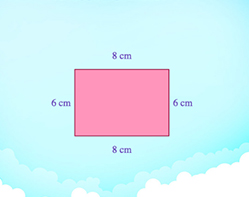Quadrilaterals are closed figures with 4 sides. Identify different quadrilaterals like rectangles, squares, rhombuses and parallelograms.

Covers Common Core Curriculum 3.G.1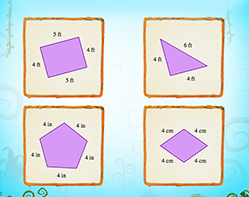A square is a square, even if you turn it upside down! Identify quadrilaterals presented in different orientations.

Covers Common Core Curriculum 3.G.1

In geometry, a quadrilateral can be defined as a closed,  two-dimensional shape which has four straight sides

The polygon has four vertices or corners.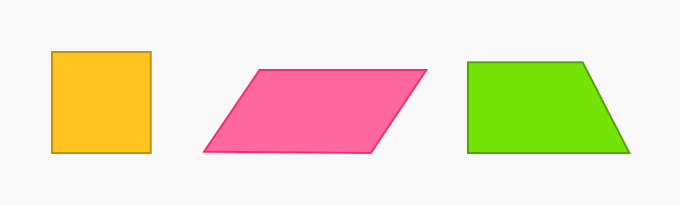We can find the shape of quadrilaterals in various things around us, like in a chess board, a deck of cards, a kite, a tub of popcorn, a sign board and in an arrow.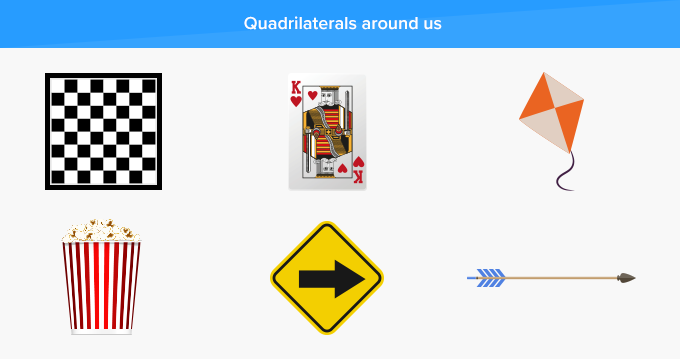• A quadrilateral has 4 sides, 4 angles and 4 vertices.
• A quadrilateral can be regular or irregular.
• The sum of all the interior angles of a quadrilateral is 360°.

Quadrilaterals can be classified into Parallelograms, Squares, Rectangles and Rhombuses. Square, Rectangle and Rhombus are also Parallelograms.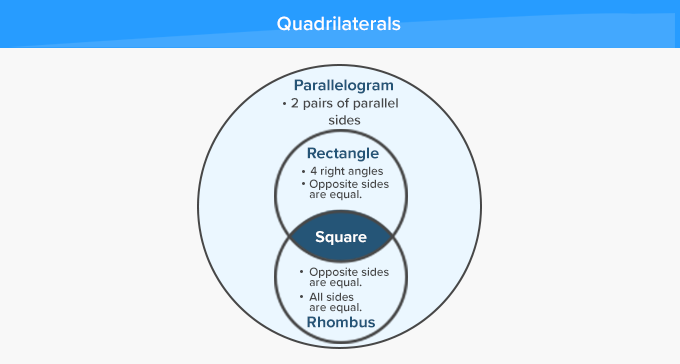Here’s a list of the types of quadrilaterals with their name, pictures and properties:

 Name of the Quadrilateral: Picture of Quadrilateral: Properties of the Quadrilateral: Parallelogram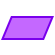Opposite sides are parallel.  Opposites sides are equal.  Opposite angles are equal. Square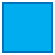All sides are equal.  All angles are equal and measure 90°. Rectangle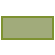Opposite sides are parallel.  Opposites sides are equal.  All angles are equal and measure 90°. Rhombus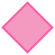All sides are equal.  Opposite angles are equal. Trapezoid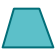Opposite sides are parallel.  Adjacent angles add up to 180°.

 Fun Facts The word quadrilateral has originated from two Latin words quadri which means “four” and, latus meaning “side”.

###Let's sing!

Four sides and, so are the corners four,
Find a quadrilateral on the bathroom floor.
You may see another in the wooden door,
Or in the boat and oars, near the seashore!!

###Let's do it!

Instead of handing out quadrilateral coloring worksheets to your children, ask them to observe and note the things in the shape of regular and irregular quadrilaterals, such as in a television, a laptop, a book or in a kite. Further, you can discuss and show videos to review the properties of different quadrilaterals.

###Related math vocabulary

Won Numerous Awards & Honors